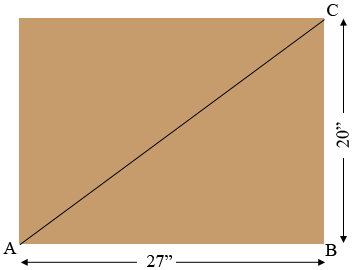SEARCH HOMEMath Central Quandaries & QueriesQuestion from Aaron: I want to make a table with a puzzle embedded in it. The table top would be a 36" circle and the puzzle is 20"x27" I'm thinking that it's not going to fit, but not sure. Any help would be appreciated. Thanks, AaronHi Aaron,

It's surprising how many times Pythagoras Theorem is helpful.Triangle $ABC$ is a right triangle and hence Pythagoras Theorem tells us that

$|AB|^2 + |BC|^2 = |CA|^2$

or

$|CA|^2 = 27^2 + 20^2 = 1129$

so

$|CA| = \sqrt{1129} = 33.6 \mbox{ inches.}$

Thus it will fit but it is tight. Each corner of the puzzle will only be 1.8 inches from the circle.

PennyMath Central is supported by the University of Regina and the Imperial Oil Foundation.